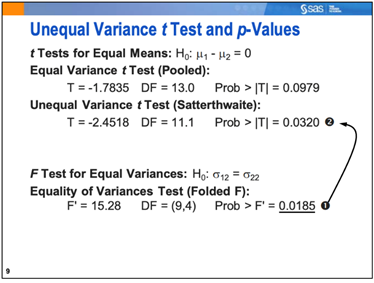T-Test

Example Codes:

Objective

Use t-test to compare the difference of a continuous variable between two groups. You need to verify the assumptions of a two-sample t-test.

Here is the general form of t-test:

PROC TTEST DATA=SAS-data-set;

CLASS variable;

VAR variables;

PAIRED variable1*variable2;

RUN;

CLASS specifies the two-level variable for the analysis. Only one variable is allowed in the CLASS statement. VAR specifies numeric response variables for the analysis. If the VAR statement is not specified, PROC TTEST analyzes all numeric variables in the input data set that are not listed in a CLASS (or BY) statement. PAIRED specifies pairs of numeric response variables from which difference scores (variable1-variable2) are calculated. A one-sample t-test is then performed on the difference scores. If the CLASS statement and PAIRED statement are omitted, PROC TTEST performs a one-sample t-test. When the CLASS statement is present, a two-sample test is performed. When a PAIRED statement is present instead, a paired t-test is performed.

Case 1 - equal variance

If the p-value is greater than the significant level, it is the test that the variance is equal. Then we use the equal variance t-test (pooled) results as shown.Case 2 - unequal variance

If the p-value less than the significant level, it is the test that variance is unequal. Then we use the unequal variance t-test (Satterthwaite) results as shown.Example Code in SAS

proc ttest data=sasuser.TestScores plots(shownull)=interval;

class Gender;

var SATScore;

run;

Example Code in SAS

DATA UNPAIRED ;

INPUT COLOUR \$ RTIME @@ ;

DATALINES ;

GREEN 232.6    RED 232.0

GREEN 257.5    RED 250.5

GREEN 253.1    RED 237.1

GREEN 205.4    RED 201.5

GREEN 226.0    RED 211.1

RUN ;

DATA PAIRED  ;

INPUT   RTIMEG   RTIMER  @@ ;

DIFFRT = RTIMEG  -  RTIMER  ;

DATALINES ;

232.6    232.0

257.5    250.5

253.1    237.1

205.4    201.5

226.0    211.1

RUN ;

PROC SORT DATA = UNPAIRED ; BY COLOUR ; RUN ;

PROC MEANS   DATA = UNPAIRED   N   MEAN   VAR STD   STDERR MAXDEC = 3  ;

BY COLOUR ;

VAR   RTIME ;

RUN ;

PROC    TTEST    DATA = UNPAIRED ;

CLASS   COLOUR ;   VAR   RTIME ;   RUN ;

PROC  TTEST  DATA = PAIRED;  PAIRED  RTIMEG * RTIMER ;  RUN;

Example Code in R

unpaired = data.frame(

colour = c(rep("GREEN", 5), rep("RED", 5)),

rtime = c(232.6, 257.5, 253.1, 205.4, 226.0, 232.0, 250.5, 237.1, 201.5, 211.1)

)

paired = data.frame(

rtimeg = c(232.6, 257.5, 253.1, 205.4, 226.0),

rtimer = c(232.0, 250.5, 237.1, 201.5, 211.1)

)

# Unpaired

t.test(unpaired\$rtime ~ unpaired\$colour, var.equal = TRUE)

# Paired

t.test(paired\$rtimeg, paired\$rtimer, paired = TRUE)